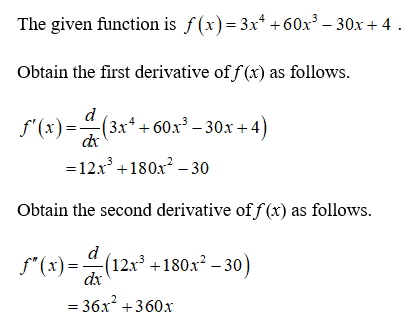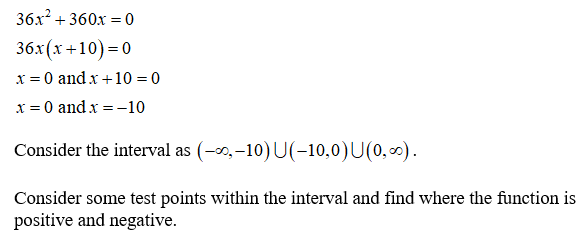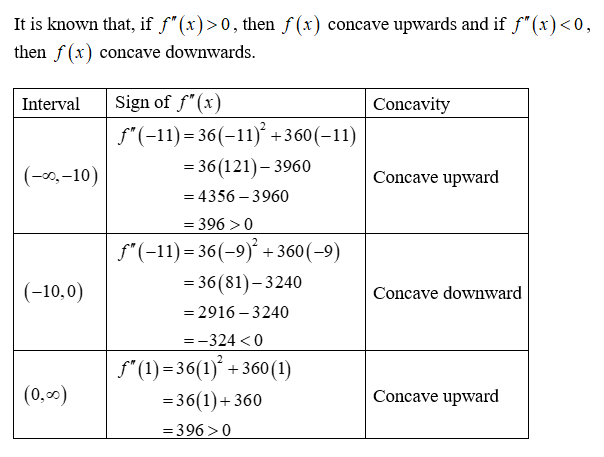# Consider the equation below.f(x) = 3x4 + 60x3 − 30x + 4 (a) Give the intervals where f(x) is concave down. (Enter your answer using interval notation. If an answer does not exist, enter DNE.)(b) Give the interval) where f(x) is concave up. (Enter your answer using interval notation. If an answer does not exist, enter DNE.)(c) Find the x-coordinates of the inflection points for f(x). (Enter your answers as a comma-separated list. If an answer does not exist, enter DNE.)x =

Question
4 views

Consider the equation below.

f(x) = 3x4 + 60x3 − 30x + 4

(a) Give the intervals where f(x) is concave down. (Enter your answer using interval notation. If an answer does not exist, enter DNE.)

(b) Give the interval) where f(x) is concave up. (Enter your answer using interval notation. If an answer does not exist, enter DNE.)

(c) Find the x-coordinates of the inflection points for f(x). (Enter your answers as a comma-separated list. If an answer does not exist, enter DNE.)

x =

check_circle

star
star
star
star
star
1 Rating
Step 1Step 2

Equate the second derivative to 0 and obtain the inflection point.Step 3...

### Want to see the full answer?

See Solution

#### Want to see this answer and more?

Solutions are written by subject experts who are available 24/7. Questions are typically answered within 1 hour.*

See Solution
*Response times may vary by subject and question.
Tagged in